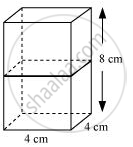Share

# 2 cubes each of volume 64 cm^3 are joined end to end. Find the surface area of the resulting cuboid. - CBSE Class 10 - Mathematics

ConceptSurface Area of a Combination of Solids

#### Question

2 cubes each of volume 64 cm3 are joined end to end. Find the surface area of the resulting cuboid.

#### Solution

Given that,

Volume of cubes = 64 cm3

(Edge) 3 = 64

Edge = 4 cmIf cubes are joined end to end, the dimensions of the resulting cuboid will be 4 cm, 4 cm, 8 cm.

∴Surface area of cuboids = 2(lb+bh+lh)

= 2(4x4+4x8+4x8)

=2(16+32+32)

2(16+64)

=2x80 = 160 cm2

Is there an error in this question or solution?

#### APPEARS IN

NCERT Solution for Mathematics Textbook for Class 10 (2019 to Current)
Chapter 13: Surface Areas and Volumes
Ex. 13.10 | Q: 1 | Page no. 244

#### Video TutorialsVIEW ALL 

Solution 2 cubes each of volume 64 cm^3 are joined end to end. Find the surface area of the resulting cuboid. Concept: Surface Area of a Combination of Solids.
S